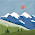## 2012年12月22日

### UVa 11513 - 9 Puzzle

```#include <cstdio>
#include <cstdlib>
#include <queue>
#include <string>
#include <map>
using namespace std;

int main() {
int i, tmp;
map<int, string> visit; // every integer(key) has its string(value) http://www.cplusplus.com/reference/map/map/
int now = 123456789; // first state
visit[now] = ""; // path is NULL
queue<int> q; // queue of BFS
q.push(now); // push first state in queue

// BFS
while (!q.empty()) {
now = q.front();
string path = visit[now];
q.pop();
int index;
string nextP;
for (i = 0; i < 3; i++) { // loop that swaps every row
sprintf(nextV, "%d", now); // print this state on a string "nextV", it'll make it easy to do swap
nextP = path;

// shift this row
index = i * 3 + 2;
tmp = nextV[index - 2];
nextV[index - 2] = nextV[index - 1];
nextV[index - 1] = nextV[index];
nextV[index] = tmp;

if (visit.count(atoi(nextV))) continue; // if we've reached this state, then do nothing

// add "H + row number" after path
add = i + 1 + '0';

q.push(atoi(nextV)); // push this state in BFS queue
visit[atoi(nextV)] = nextP; // stored string of this path
}
for (i = 0; i < 3; i++) { // loop that swaps every column
sprintf(nextV, "%d", now); // print this state on a string "nextV", it'll make it easy to do swap
nextP = path;

// shift this column
tmp = nextV[i + 6];
nextV[i + 6] = nextV[i + 3];
nextV[i + 3] = nextV[i];
nextV[i] = tmp;

if (visit.count(atoi(nextV))) continue; // if we've reached this state, then do nothing

// add "V + column number" after path
add = i + 1 + '0';
q.push(atoi(nextV));
visit[atoi(nextV)] = nextP;
}
}
// After this BFS algorithm, we've generate all possible state which can reach from 123456789

while (scanf("%d", &tmp) && tmp) {
int ans = tmp;
for (i = 1; i < 9; i++) {
scanf("%d", &tmp);
ans = ans * 10 + tmp;
}

bool find = visit.count(ans); // return true if we can find this state

if (find) {
string path = visit[ans]; // path of this state was stored in map
printf("%d ", path.length() >> 1); // length of moving is length of this path / 2

// because we generate solution from 123456789,
// but now we want to reach 123456789,
// so we have to print the answer in contrary direction
for (i = path.length() - 2; i >= 0; i -= 2)
printf("%c%c", path[i], path[i + 1]);
puts("");
} else {
puts("Not solvable");
}
}
return 0;
}
```

#### 4 則留言:

1.Hello

I am pretty puzzled about how to solve this problem. I am not pretty familiar with C++ so even though the code is right above, can you still please explain it? I would be very thankful if you can help!

btw, I am from Taiwan,too XD, and I am also currently learning English(well not pretty active) and programming(more active due to having a programming class).

2.Hi, nice to meet you, I'll try my best to explain in English. XD

This is a BFS algorithm.

I used int to present my state, every state has a path, and this path is present in a string.
This is what visit in line 10. do, and map in C++ just like a hash
function, map means every integer(key) in this map has its string(value).

First, I generate all possible state which can reach from 123456789 from line 15. to line 53., and the first loop (line 22. to line 37.) moves every row, the second loop (line 38. to 52.) moves every column. After each move, you have to check if you've visited this state before, this is why

if (visit.count(atoi(nextV))) continue;

And after this BFS algorithm, we have all possible solution from 123456789

bool find = visit.count(ans);

means if I could reach this solution from 123456789, then I'll print the path that I stored in visit, else means no solution.

1.3.